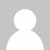OK, here’s my solution to Advent of Code day 3:

C# part a (using Scan from MoreLINQ):

``````File.ReadAllText("day3.txt")
.Scan(new { x = 0, y = 0 }, (state, c) =>
c == '>' ? new { x = state.x + 1, y = state.y } :
c == '^' ? new { x = state.x, y = state.y + 1 } :
c == '<' ? new { x = state.x - 1, y = state.y } :
new { x = state.x, y = state.y - 1 })
.Select(p => String.Format("{0},{1}", p.x, p.y))
.GroupBy(p => p)
.Count()
``````

C# part b:

``````void Main()
{
.Batch(2)
.Scan(new { Santa = new Pos(0, 0), RoboSanta = new Pos(0, 0) }, (state, c) =>
new { Santa = Move(c.First(), state.Santa),
RoboSanta = Move(c.Last(), state.RoboSanta)
})
.SelectMany(p => new[] { p.Santa, p.RoboSanta } )
.Select(p => String.Format("{0},{1}", p.X, p.Y))
.GroupBy(p => p)
.Count()
.Dump();
}

public class Pos
{
public Pos(int x, int y)
{
X = x; Y = y;
}
public int X { get; }
public int Y { get; }
}

Pos Move(char direction, Pos startingPoint)
{
return
direction == '>' ? new Pos(startingPoint.X + 1, startingPoint.Y) :
direction == '^' ? new Pos(startingPoint.X, startingPoint.Y + 1) :
direction == '<' ? new Pos(startingPoint.X - 1, startingPoint.Y) :
new Pos(startingPoint.X, startingPoint.Y - 1);
}
``````

F# part a:

``````File.ReadAllText("day3.txt")
|> Seq.map (fun c -> match c with | '>' -> (1,0) | '^' -> (0,1) | '<' -> (-1,0) | _ -> (0,-1))
|> Seq.scan (fun (x1,y1) (x2,y2) -> (x1 + x2, y1 + y2)) (0,0)
|> Seq.distinct
|> Seq.length
``````

F# part b:

``````let getVector c = match c with | '>' -> (1,0) | '^' -> (0,1) | '<' -> (-1,0) | _ -> (0,-1)
let addVector (x1,y1) (x2,y2) = (x1 + x2, y1 + y2)
let directions =
let startState = ((0,0),(0,0),0)
let update (santa,roboSanta,index) nextDir =
if (index % 2) = 0 then
else

directions
|> Seq.map getVector
|> Seq.scan update startState
|> Seq.map (fun (santa,roboSanta,index) -> if index % 2 = 0 then santa else roboSanta)
|> Seq.distinct
|> Seq.length
|> Dump
``````
Want to learn more about LINQ? Be sure to check out my Pluralsight course LINQ Best Practices.You can replace the

``fun c -> match c with '>' -> //...``

by

``function '>' -> //...``

That said we have the same logic ; just written differently (I use a set to replace your distinct)

``let input = File.ReadAllText "day03.txt"let common : char seq -> _ =  Seq.scan (fun (x, y) -> function    '^' -> x, y - 1  | '>' -> x + 1, y  | 'v' -> x, y + 1  | _   -> x - 1, y) (0, 0)  >> setlet part1 () = common input |> Set.countlet part2 () =  let santa, robot =    input    |> Seq.foldBack (fun c (santa, robot) -> robot, c :: santa) <| ([], [])  common santa + common robot  |> Set.count``

The other difference is for separating santa to robot-santa ; I insert items in two list swapping them after each insertion (effectively getting odd/even separation)

Sehnsuchtnice, didn't know about set - that will come in handy for today's one. might refactor if I get a chance

Mark Heathhow to use Scan in Linqpad?
I did some search and i didnt find anyway to add MoreLinq to Linqpad

amalgaUnfortunately you do need a developer license to use NuGet packages with LinqPad. I think its quite reasonably priced, but for a free alternative, you can use the community edition of Visual Studio to try these problems.

Mark HeathOk i gonna try Visual Studio, Thanks alot ^^

amalgaYour c# solution for part b has a bug. If the input has an odd number of moves then using Last() for RoboSantas moves makes an uncommanded move on the last batch, which has only one item. Depending on the prior move instructions this may or may not result in the final answer being out by one.
To work around this I used
Robot = m.Skip(1).Any() ? l.Robot.Move(m.Last()) : l.Robot
Can you suggest a better way?

Steve Craneyes, good spot, it does assume the instructions will always be in pairs. Shouldn't be using Last() without checking that it is a two element batch (could insert another select turning each batch into an array, allowing checking of length)

Mark Heath